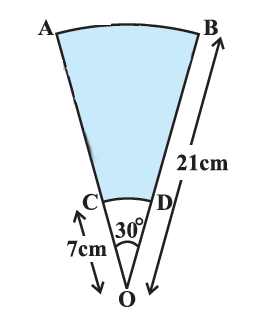# Ex.12.3 Q14 Areas Related to Circles Solution - NCERT Maths Class 10

Go back to  'Ex.12.3'

## Question

$${AB}$$ and $${CD}$$ are respectively arcs of two concentric circles of radii $$\text{21 cm}$$ and $$\text{7 cm}$$ and center $${O}$$ (see Figure). If $$\angle {AOB} = 30^\circ$$, find the area of the shaded region.Video Solution
Areas Related To Circles
Ex 12.3 | Question 14

## Text Solution

What is known?

$${AB}$$ and $${CD}$$ are arcs of two concentric circle of radii $$\text{21 cm}$$ and $$\text{7 cm}$$ respectively and centre $${O.}$$

$$\angle {{ AOB }}= \,30^\circ$$

What is unknown?

Reasoning:

Area of the shaded region $$=$$ Area of sector $${ABO}\, -$$ Area of sector $${CDO}$$

Areas of sectors $${ABO}$$ and $${CDO}$$ can be found by using the formula of

Area of sector of angle \begin{align} \theta = \frac{\theta }{{{{360}^\circ }}} \times \pi {r^2}\end{align}

where $${r}$$ is radius of the circle and angle.

with degree measure $$\theta$$

with $$\theta = {30^ \circ }$$ for the both the sector $${ABO}$$ and $${CDO}$$ angle $$\theta = {30^\circ}$$ and radii 21cm and 7cm respectively.

Steps:

Radius of the sector ABO,$$\rm{}R = OB = 21\rm{}cm$$

Radius of the sector CDO,$$\rm{}r = OD = 7\rm{}cm$$

For both the sector ABOand CDO angle,$$\theta = {90^\circ}$$

Area of shaded region $$=$$ Area of sector $${ABO}\; –$$ Area of sector $${CDO}$$

\begin{align}&= \frac{\rm{\theta }}{{360}^{\rm{o}}} \times \pi {R^2} - \frac{\rm{\theta}}{{360}^\circ } \times \pi {r^2}\\&= \frac{{\rm{\theta }}}{{{{360}^{\rm{o}}}}} \times \pi \left( {{R^2} - {r^2}} \right)\\ &= \frac{{{{30}^{\rm{o}}}}}{{{{360}^{\rm{o}}}}} \times \frac{{22}}{7}\left( {{{\left( {21cm} \right)}^2} - {{\left( {7cm} \right)}^2}} \right)\\&= \frac{1}{{12}} \times \frac{{22}}{7} \times \left( {441c{m^2} - 49c{m^2}} \right)\\&= \frac{{11}}{{42}} \times 392c{m^2}\\&= \frac{{308}}{3}c{m^2}\end{align}

Learn from the best math teachers and top your exams

• Live one on one classroom and doubt clearing
• Practice worksheets in and after class for conceptual clarity
• Personalized curriculum to keep up with school